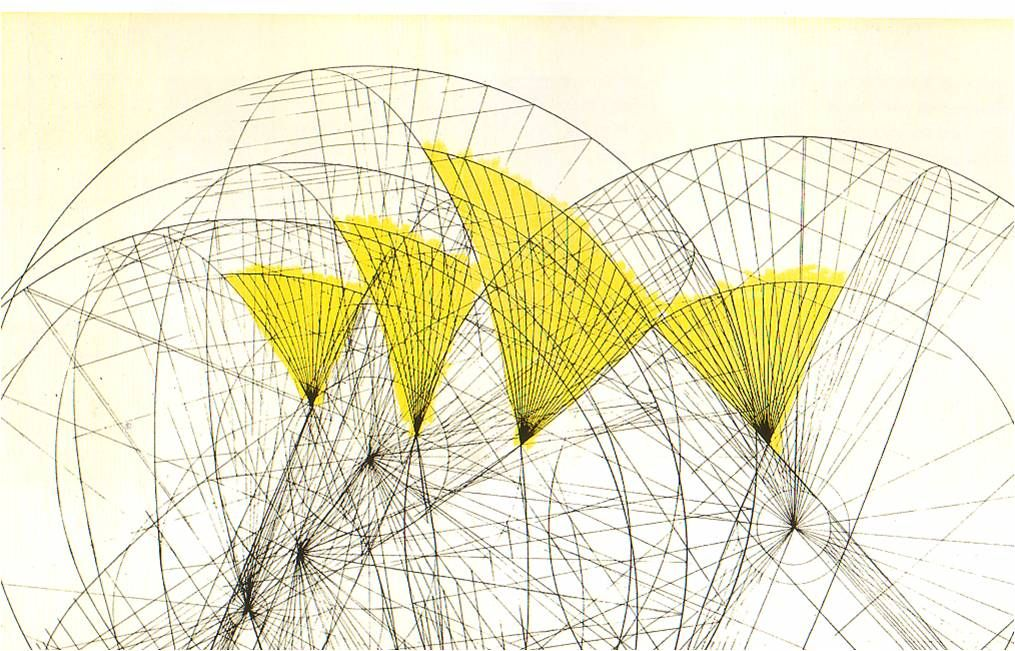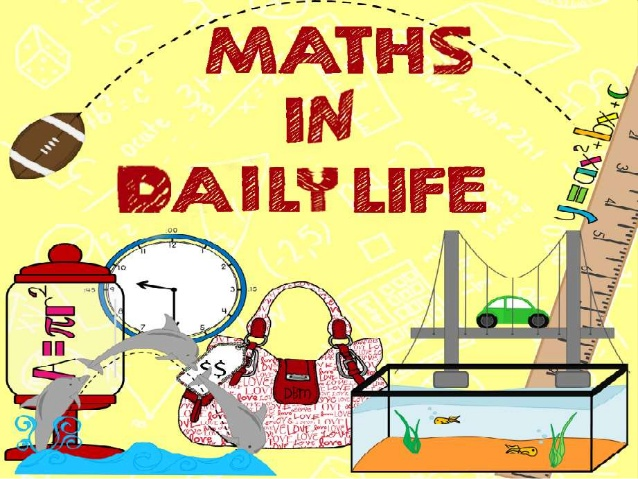## The Language of Mathematics

Mathematics consist millions of distinct equations, infinite numbers and the whole Greek alphabet! Nonetheless, mathematics is written in the exact same way in all languages of the world. In other words, a mathematical equation or expression does not need to be translated in another language to be understood by someone living on the other side of the world. Mathematics do not discriminate, in terms of religion, gender, color or language. 2 + 2 = 4 in each and every single one of the countries of the whole world. (Why Math Is Important In Life, 2018)

While usually children and teenagers complain of the difficultness and boredom caused by mathematics, a world without it, may mean that we experience a way different kind of world, a world on a much less interesting level. Imagine a world without math. An architect would not know how to calculate angles or lines, a doctor would not know how to count your heartbeat, a chemist would not be able to prepare medicines without accurately measuring the quantity, an engineer would not be able to build bridges etc. (Nautiya, 2012)

Math help us understand the world and we use the world to understand math (Understanding the World Through Math, n.d.)​Several areas of mathematics originated by attempting to describe the real world and solve the real-world phenomena. Some examples are measuring farms (geometry), falling apples (calculus) or even gambling (probability). Mathematics has been extensively successful in helping us understand in more depth the universe – from its large scales (physical cosmology) to its smallest (quantum mechanics).

In our days, mathematics can be found all around us, in every step of our lives. Have you ever thought how many things of our everyday life use mathematics and we take it for granted? From the watch and calendar to baking and sports, from electricity and technology (computers, television, mobile phones) to gardening and art. Because of mathematics, we know how everything around us works and can apply it in every field and profession. (Krishnan, 2016) Considering all of the aforementioned, people all over the world understand these concepts without the need of translating them. So, should math be categorized as a language?

According to the Italian astronomer Galileo Galilei, “[The universe] cannot be read until we have learned the language and become familiar with the characters in which it is written. It is written in mathematical language.” If mathematics is considered as a language, then as all other ordinary languages, mathematics is independent of the other and there is no need to rely on another language to be understood. (Silver, 2017)

Some key facts on why Mathematics can indeed be considered as a language:

• It is a system of communication, that has vocabulary, grammar, syntax
• The words and symbols have meaning
• Written rather than spoken form of communication
• An expression of thought in a specific way
• There is a group of people that use it and understand it (Helmenstine, 2019)Galileo Galilei

Therefore, mathematics can be viewed as a simpler, more consistent and more regular language than the English one. The numbers can represent the nouns and the operational signs can represent verbs. A mathematical equation such as ‘2 x 3 = 6’ can be thought as a sentence. Just like the English language, Mathematics are based on grammar and correct syntax. For example, from a young age, children learn that a math sentence is written as 5 + 6 = 11 and not as 5 6 + = 11. The language of mathematics has an infinity number of nouns and just five verbs (operational signs) +, -, /, x, =. (Teaching Math as a Language, 2016)

Mathematics is a language that can be more carefully defined and more profoundly abstracted than the ordinary thought and expression we use daily. It might be considered as a language; however, mathematics differ from ordinary languages in an important way – by the rules of manipulation. Once a statement is changed in a mathematical form, it can be manipulated according to the rules. Every configuration of the symbols will represent facts in harmony depending on the ones of the original statement. (“Language of Mathematics,” 2020)

Visit the project website : www.math-reality.eu

## Related project :

Tools for math teachers to add art works and hands-on exercises in their lessons.

First European training on the practices and tools in 6 main technologies involved in enhanced contents publishing and dissemination.

Bibliography:

10 Reasons Why Math Is Important In Life [Guide + Examples]. (2018). Pi Day. https://www.piday.org/10-reasons-why-math-is-important-in-life/Helmenstine, A. M. (2019).

Why Mathematics Is a Language. ThoughtCo. https://www.thoughtco.com/why-mathematics-is-a-language-4158142

Krishnan, P. (2016). Life Without Mathematics | Testprep Content Hub. https://www.meritnation.com/testprep/hub/life-without-mathematics/

Language of mathematics. (2020). In Wikipedia. https://en.wikipedia.org/w/index.php?title=Language_of_mathematics&oldid=970110907

Nautiya, S. (2012). Life without mathematics. /en/article/life-without-mathematics

Silver, D. S. (2017). The New Language of Mathematics. American Scientist. https://www.americanscientist.org/article/the-new-language-of-mathematics

Teaching Math as a Language. (2016). Landmark Outreach. https://www.landmarkoutreach.org/strategies/math-as-a-language/

Understanding the World Through Math. (n.d.). Asia Society. Retrieved July 29, 2020, from https://asiasociety.org/education/understanding-world-through-math